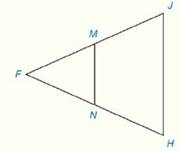Chapter 4.CR, Problem 23CR### Elementary Geometry for College St...

6th Edition
Daniel C. Alexander + 1 other
ISBN: 9781285195698

#### Solutions

Chapter
Section### Elementary Geometry for College St...

6th Edition
Daniel C. Alexander + 1 other
ISBN: 9781285195698
Textbook Problem
1 views

# Review ExercisesIn Review Exercises 22 to 24, M and N are the midpoints of F J - and F H - respectively.Given: J H = 12 m ∠ J = 80 ° m ∠ F = 60 ° Prove: M N ,   m ∠ F M N ,   m ∠ F N M

To determine

To Find:

The length of the line joining the mid points of the sides of the triangle and measure of angles based on the information provided.

Explanation

Formula Used:

Sum of the interior angle of a triangle is 180°.

Theorem on triangle:

The line segment that joins the midpoints of two sides of a triangle is parallel to the third side and has a length equal to one-half the length of the third side.

That is, ABC with midpoints M and N of AB- and AC-, respectively. Then MN-BC-. It can be concluded that BC=12(MN)

Calculation:

It is given that FJH is an isosceles triangle with M and N are the midpoints of FJ- and FH- respectively.

Also given that JH=12, mJ=80° and mF=60°.

Applying the theorem, MN is parallel to JH.

So mFHJ=mFNM and mFJH=mFMN.

Here mJ=80°

### Still sussing out bartleby?

Check out a sample textbook solution.

See a sample solution

#### The Solution to Your Study Problems

Bartleby provides explanations to thousands of textbook problems written by our experts, many with advanced degrees!

Get Started

#### Find more solutions based on key concepts